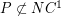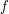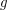## Понедельник 26 сентября. "An Information Complexity Approach to the KRW Composition Conjecture"

Понедельник, 26 сентября, 402. Начало в 15:00.

Докладчик: Александр Смаль.

Тема: An Information Complexity Approach to the KRW Composition Conjecture.

### Abstract

На семинаре будет рассказано о результатах из статьи Dmitry Gavinsky, Or Meir, Omri Weinstein, Avi Wigderson "Toward Better Formula Lower Bounds: An Information Complexity Approach to the KRW Composition Conjecture".

Abstract

One of the major open problems in complexity theory is proving super-logarithmic lower bounds on the depth of circuits (i.e.,). This problem is interesting for two reasons: first, it is tightly related to understanding the power of parallel computation and of small-space computation; second, it is one of the first milestones toward proving super-polynomial circuit lower bounds.

Karchmer, Raz, and Wigderson [KRW95] suggested to approach this problem by proving the following conjecture: given two Boolean functionsand, the depth complexity of the composed function gf is roughly the sum of the depth complexities ofand. They showed that the validity of this conjecture would imply that.

As a starting point for studying the composition of functions, they introduced a relation called "the universal relation", and suggested to study the composition of universal relations. This suggestion proved fruitful, and an analogue of the KRW conjecture for the universal relation was proved by Edmonds et. al. [EIRS01]. An alternative proof was given later by Håstad and Wigderson [HW93]. However, studying the composition of functions seems more difficult, and the KRW conjecture is still wide open.

In this work, we make a natural step in this direction, which lies between what is known and the original conjecture: we show that an analogue of the conjecture holds for the composition of a function with a universal relation.